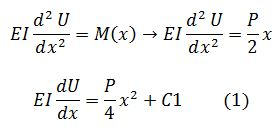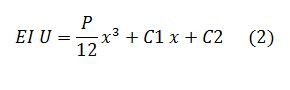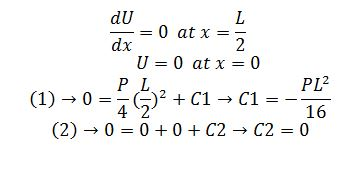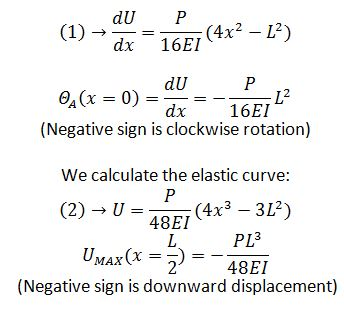Friday, 10 February 2017 10:54cat

## Calculation Example - Calculate the equation of the elastic curve. Featured

Written by  TheStructuralEngineer.info

Calculate the equation of the elastic curve .Determine the pinned beam’s maximum deflection. EI constant.

Solution

From the previous exercise (Calculation example-Calculate member diagrams) published (17 January 2017), we work for the section 0<x<L/2.

The Shear force is S(x)= P/2

The Moment is M(x)= P/2 x

The Slope and the Elastic Curve are:Due to symmetry, boundary conditions are:We calculate the slope: